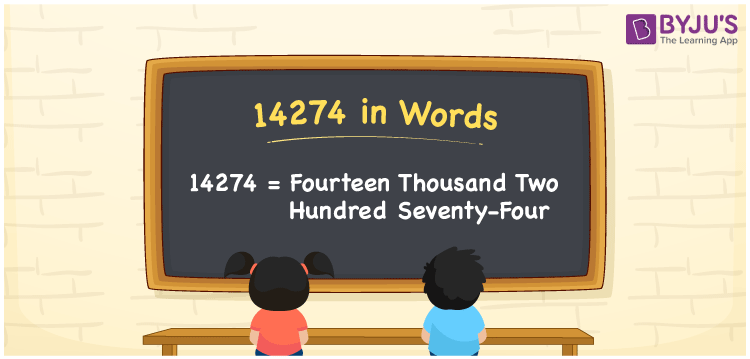# 14274 in Words

The number 14274 in words is “fourteen thousand two hundred seventy-four”. The numeral 14274 is a five-digit number and its place values are useful to write the number name of 14274. Also, the natural number 14274 exists between 14273 and 14275. In this article, we are going to learn the process of writing the number 14274 in words using the place value system.

 14274 in Words: Fourteen Thousand Two Hundred Seventy-four. Fourteen Thousand Two Hundred Seventy-four in Numerical Form: 14274.

## 14274 in English Words## How to Write 14274 in Words?

Go through the place value table for the numeral 1424 and write the number 14274 in words.

 Ten-thousands Thousands Hundreds Tens Ones 1 4 2 7 4

The expanded form of 14274 is as follows:

= 1 × Ten thousand + 4 × Thousand + 2 × Hundred + 7 × Ten + 4 × One

= 1 × 10000 + 4 × 1000 + 2 × 100 + 7 × 10 + 4 × 1

= 10000 + 4000 + 200 + 70 + 4

= 14274

= Fourteen thousand two hundred seventy-four

Hence, 14274 in words is fourteen thousand two hundred seventy-four.

14274 in words – Fourteen thousand two hundred seventy-four

Is 14274 an odd number? – No

Is 14274 an even number? – Yes

Is 14274 a perfect square number? – No

Is 14274 a perfect cube number? – No

Is 14274 a prime number? – No

Is 14274 a composite number? – Yes

## Frequently Asked Questions on 14274 in Words

Q1

### How do you write 14274 in words?

14274 in words is fourteen thousand two hundred seventy-four.

Q2

### Simplify 14000 + 274, and express it in words.

Simplifying 14000 + 274, we get 14274. Hence, 14274 in words is fourteen thousand two hundred seventy-four.

Q3

### Is 14274 an even number?

Yes, 14274 is an even number.